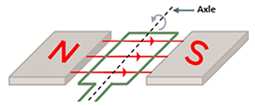# Magnetic Flux Formula

## Magnetic Flux Formula - Definitions & Practice Questions

Magnetic flux refers to the total number of magnetic field lines penetrating any surface placed perpendicular to the magnetic field. It is calculated as the product of the average magnetic field strength and the perpendicular area it penetrates.
Magnetic flux is denoted by ΦB where B represents magnetic field, and its unit is tesla-metre2 or weber (Wb).
Mathematically, ${\phi _B} = \vec B\,.\,\vec A$
Or, ${\phi _B} = BA\cos \theta$
Where, B is the magnetic field, A is the surface area and $\theta$is the angle between the normal to the surface and the magnetic field.
If a coil of n turns and area of cross section A is placed in a magnetic field of strength B, then the total flux associated with the coil is:
${\phi _B} = n\,BA\cos \theta$

Example:
A magnetic field of 2.5 T passes perpendicular to a disc of radius 2 cm. Find the magnetic flux associated with the disc.
Solution:
B = 2.5 T, r = 2 cm = 2 × 10–2 m, $\theta$= 0, ${\phi _B}$=?
${\phi _B} = BA\cos \theta = 2.5 \times \pi {(2 \times {10^{--2}})^2} \times \cos 0 = 3.14 \times {10^{--3}}Wb$

Example:
A coil of area of cross section 10–2 m2 and 100 turns is placed in a magnetic field of strength 1 T, with its axis making an angle 60° with the field. Find the total flux associated with the field.
Solution:
B = 1 T, A = 10–2 m2, $\theta$= 60°, n = 100, ${\phi _B}$=?
${\phi _B} = n\,BA\cos \theta = 100 \times 1 \times {10^{--2}} \times \cos 60^\circ = 0.5\,Wb$

Question: When the coil is rotated between the pole pieces of a magnet as shown, during one complete rotation of the coil, how often will the magnetic flux linked with coil be maximum and minimum?Options:
(a) maximum and minimum once each,
(b) maximum and minimum twice each
(c) maximum once, minimum twice
(d) maximum twice, minimum once